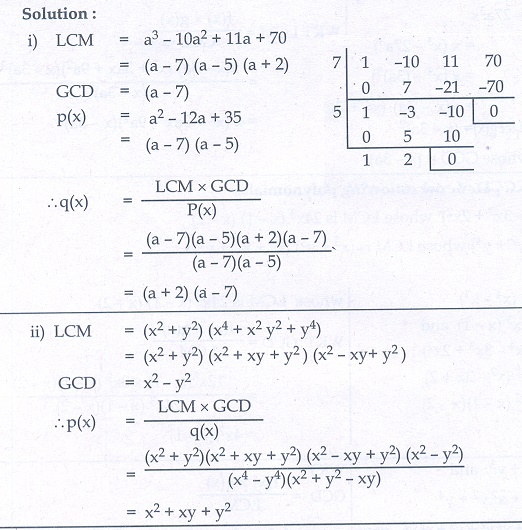Home | | Maths 10th Std | Exercise 3.3: Relationship between LCM and GCD

# Exercise 3.3: Relationship between LCM and GCD

Maths Book back answers and solution for Exercise questions - Mathematics : Algebra: Relationship between LCM and GCD: Exercise Problem Questions with Answer

Exercise 3.3

1. Find the LCM and GCD for the following and verify that f (x)× g (x) = LCM ×GCD

(i) 21x 2y, 35xy2

(ii) (x3  − 1)(x + 1), (x3  + 1)

(iii) (x2y + xy2 ), (x2  + xy)2. Find the LCM of each pair of the following polynomials

(i) 2  + 4−12, a 2  5+ 6 whose GCD is a  2

(ii) 4 - 273x, (- 3a)2  whose GCD is (- 3a)3. Find the GCD of each pair of the following polynomials

(i) 12(x 4 - x 3 ),  8(x 4 − 3x 3 + 2x 2 ) whose LCM is 24x 3(x - 1)(x - 2)

(ii) (x 3  + y3 ), (x 4  + x 2y 2  + y4 ) whose LCM is (x 3  + y 3 )(x 2  + xy + y2 )4. Given the LCM and GCD of the two polynomials p(x) and q(x) find the unknown polynomial in the following table1. (i) 105x 2y2 , 7xy

(ii) (x  1)(x + 1)(x 2  + x + 1)(x 2  x + 1) , (x + 1)

(iii) xy(+ y) , x (+ y)

2.(i) (a + 6) (a - 2) (a - 3)

(ii) ( 3)2 (3ax + 9a2 )

3.(i) 4x 2 (x -1)

(ii) 2  xy + y2

4 (i) (+ 2)( 7)

(ii) 2  + xy + y2

Tags : Problem Questions with Answer, Solution | Mathematics , 10th Mathematics : UNIT 3 : Algebra
Study Material, Lecturing Notes, Assignment, Reference, Wiki description explanation, brief detail
10th Mathematics : UNIT 3 : Algebra : Exercise 3.3: Relationship between LCM and GCD | Problem Questions with Answer, Solution | Mathematics